How to Learn in 24 Hours?The Rapid Learning Movie

 Need Help? M-F: 9am-5pm(PST): Toll-Free: (877) RAPID-10 US Direct: (714) 692-2900 Int'l: 001-714-692-2900 24/7 Online Technical Support: The Rapid Support Center Secure Online Order:Got Questions? Frequently Asked Questions
 Need Proof? Testimonials by Our Users
 Trustlink is a Better Business Bureau Program. Rapid Learning Center is a fivr-star business. External TrustLink Reviews

 Rapid Learning Courses: MCAT in 24 Hours (2015-16) USMLE in 24 Hours (Boards) Chemistry in 24 Hours Biology in 24 Hours Physics in 24 Hours Mathematics in 24 Hours Psychology in 24 Hours SAT in 24 Hours ACT in 24 Hours AP in 24 Hours CLEP in 24 Hours DAT in 24 Hours (Dental) OAT in 24 Hours (Optometry) PCAT in 24 Hours (Pharmacy) Nursing Entrance Exams Certification in 24 Hours eBook - Survival Kits Audiobooks (MP3)

 Tell-A-Friend: Have friends taking science and math courses too? Tell them about our rapid learning system.Home »  Mathematics »  Pre-Calculus

Basic Graphs and Graphical Representation of Data

 Topic Review on "Title": Coordinate systems:  They are two mutually perpendicular coordinate lines which intersect in the origin O on each line. The four quadrants in a coordinate system: Quadrant I is the area where a>0 and b>0. Quadrant II is the area where a<0 and b>0. Quadrant III is the area where a<0 and b<0. Quadrant IV is the area where a>0 and b<0. Distance:  The distance between any two points is defined as:. Data sets: The grouping or collection of similar or related data. Subsets: If A and B are sets and every element of A is also an element of B, then: A is a subset of B, denoted by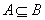. Product of two data sets: If S and T are sets, the product set of S and T will be denoted by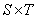, which satisfies. Line: A geometric figure formed by a point moving along a fixed direction and the reverse direction. Slope of a line: If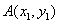and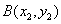are arbitrary two points on the line, the slope can be calculated by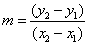. Midpoint of any two points: The distance between two arbitrary points is given by.

Rapid Study Kit for "Title":
 Flash Movie Flash Game Flash Card Core Concept Tutorial Problem Solving Drill Review Cheat Sheet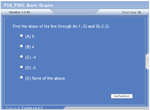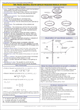"Title" Tutorial Summary : This tutorial describes basic graphs of functions and how they are graphically represented in the Cartesian plane. The coordinate systems in two variables are introduced with the help of graphs. Key concepts such as the midpoint, and the distance of between two points are discussed in this tutorial. The use of relations between two sets of points and their graphs is introduced so data can be graphically represented.

 Tutorial Features: Specific Tutorial Features: • Step by step examples showing data sets, product of two data sets and relations are shown in this tutorial. • Examples displaying how to find the distance and midpoints between any two points are presented with the use of graphs. Series Features: • Concept map showing inter-connections of new concepts in this tutorial and those previously introduced. • Definition slides introduce terms as they are needed. • Visual representation of concepts • Animated examples—worked out step by step • A concise summary is given at the conclusion of the tutorial.

 "Title" Topic List: Coordinate Systems in two dimensions Definition of coordinate systems Definition of the four quadrantsDefinition of distance and midpointRelations Definition of data sets Subsets The product of two data sets The relation of two data setsLine in a plane Definition of a line Definition of the slope of a line Parallel and perpendicular lines

See all 24 lessons in Pre-Calculus, including concept tutorials, problem drills and cheat sheets:
Teach Yourself Pre-Calculus Visually in 24 Hours